Next: Language modeling versus other Up: The query likelihood model Previous: Estimating the query generation   Contents   Index

## Ponte and Croft's Experiments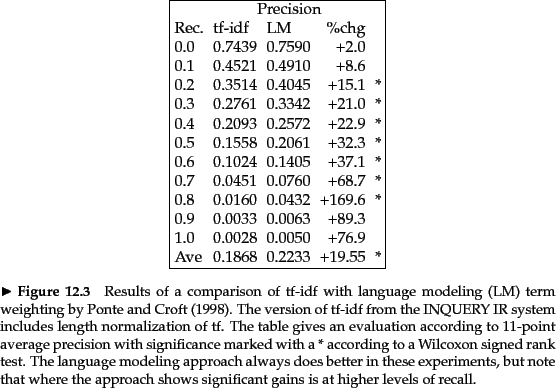Ponte and Croft (1998) present the first experiments on the language modeling approach to information retrieval. Their basic approach is the model that we have presented until now. However, we have presented an approach where the language model is a mixture of two multinomials, much as in (Miller et al., 1999, Hiemstra, 2000) rather than Ponte and Croft's multivariate Bernoulli model. The use of multinomials has been standard in most subsequent work in the LM approach and experimental results in IR, as well as evidence from text classification which we consider in Section 13.3 (page), suggests that it is superior. Ponte and Croft argued strongly for the effectiveness of the term weights that come from the language modeling approach over traditional tf-idf weights. We present a subset of their results in Figure 12.4 where they compare tf-idf to language modeling by evaluating TREC topics 202-250 over TREC disks 2 and 3. The queries are sentence-length natural language queries. The language modeling approach yields significantly better results than their baseline tf-idf based term weighting approach. And indeed the gains shown here have been extended in subsequent work.

Exercises.

• Consider making a language model from the following training text:
the martian has landed on the latin pop sensation ricky martin
1. Under a MLE-estimated unigram probability model, what are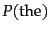and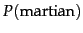?

2. Under a MLE-estimated bigram model, what are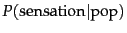and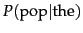?

• Suppose we have a collection that consists of the 4 documents given in the below table.
 docID Document text 1 click go the shears boys click click click 2 click click 3 metal here 4 metal shears click here
Build a query likelihood language model for this document collection. Assume a mixture model between the documents and the collection, with both weighted at 0.5. Maximum likelihood estimation (mle) is used to estimate both as unigram models. Work out the model probabilities of the queries click, shears, and hence click shears for each document, and use those probabilities to rank the documents returned by each query. Fill in these probabilities in the below table:
 Query Doc 1 Doc 2 Doc 3 Doc 4 click shears click shears
What is the final ranking of the documents for the query click shears?

• Using the calculations in Exercise 12.2.3 as inspiration or as examples where appropriate, write one sentence each describing the treatment that the model in Equation 102 gives to each of the following quantities. Include whether it is present in the model or not and whether the effect is raw or scaled.
1. Term frequency in a document
2. Collection frequency of a term
3. Document frequency of a term
4. Length normalization of a term

• In the mixture model approach to the query likelihood model (Equation 104), the probability estimate of a term is based on the term frequency of a word in a document, and the collection frequency of the word. Doing this certainly guarantees that each term of a query (in the vocabulary) has a non-zero chance of being generated by each document. But it has a more subtle but important effect of implementing a form of term weighting, related to what we saw in Chapter 6 . Explain how this works. In particular, include in your answer a concrete numeric example showing this term weighting at work.Next: Language modeling versus other Up: The query likelihood model Previous: Estimating the query generation   Contents   Index